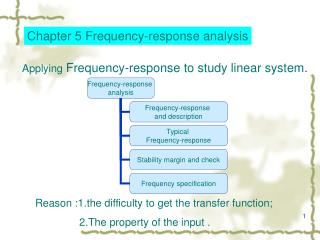DownloadDownload PresentationChapter 5 Frequency-response analysis

# Chapter 5 Frequency-response analysis

Download Presentation## Chapter 5 Frequency-response analysis

- - - - - - - - - - - - - - - - - - - - - - - - - - - E N D - - - - - - - - - - - - - - - - - - - - - - - - - - -
##### Presentation Transcript

1. Chapter 5 Frequency-response analysis Applying Frequency-response to study linear system. Reason :1.the difficulty to get the transfer function; 2.The property of the input .

2. characteristics （1）The frequency response has clear and definite physical meaning, it can be determined by experiment method. To the system difficult to write the differential equation, it has actual meaning of importance. (2) because the frequency response analyzes system by open -loop frequency characteristic, it is direct,simple, has little calculation. (3) the frequency response not only is applicable to the linear time-invariant system, but also the system with pure delay and part non-linear system.

3. 5.1Frequency-response and description 5.1.1Frequency-response basic concept Frequency characteristic,also called Frequency response，is the response characteristic to sine signal of a system or component. The output amplitude and phase generally differ from input, and vary with different input signal frequency .

4. red—input，blue—complete response，black—steady-state response 6 yss(t) amplitude 4 2 0 u(t) -2 -4 red—input，blue—complete response，black—steady-state response -6 2 y(t) -8 1.5 0 1 2 3 4 5 6 t/s yss(t) 1 amplitude 0.5 0 -0.5 -1 -1.5 y(t) u(t) -2 0 1 2 3 4 5 6 t/s

5. Assume system TF ,its Laplace transformation Given input A is a constant，output: (5-1) poles G(s) To a stable system (5-2)

6. (5-2) Coefficient to be determined (5-4) 0,when (5-5) (5-6) Owing to is a complex vector，can be expressed (5-7)

7. Amplitude-frequency characteristic (5-11) phase-frequency characteristic Steady-state output of a linear systemis a sine signal and its frequency is the same as input sine signal frequency. the amplitude ratio of output to input is note Phase deviationof output to input

8. As a case,see R-C circuit。Fig.5-3. TF is Set input (5-15) where

9. --------frequency characteristic of RC circuit 。 connected with construction and parameters of RC circuit, independent of the input signal。 , output/input amplitude ratio when steady state , output/input phase deviation when steady state because and Are the function of input signal frequency They are called respectively amplitude-frequency characteristic and phase-frequency characteristic。 Physical meaning:the output/input ratio,Or output/input amplitude ratio and output/input phase deviation . The relation between input and output when a sine signal with a frequency is exerted to a circuit.

10. Circuit output/input amplitude ratio (a) amplitude-frequency characteristic

11. Circuit output/input phase deviation (b) phase-frequency characteristic。

12. compare phase-frequency characteristic and TF is of very similar form.。

13. 5.1.2 expressed forms (1)Bode diagram or logarithmic plot (2)Polar plot (3)Log-magnitude versus phase plot Logarithmic amplitude-frequency characteristic logarithmic frequency characteristic Logarithmic phase-frequency characteristic () The longitudinal coordinate is marked according to linearity Horizontal coordinateis marked according to

14. BODE diag.

15. Polar plot,also amplitude-phase frequency characteristic . Can be expressed by a vector with amplitude and phase ， Amplitude and phase of When input signal frequency the vector G(jw) vary,the curve which its end point traces in complex plane is called polar diagram---- Nyquist curve----- Nyquist diag. Nyquist analysed feedback control system stability in 1932.

16. Nyquist DIAG.

17. 5.2typical component bode diag. 5.2.1 proportion K Please look at the following page

18. Number- decibel conversion

19. Diag.5-7 Number- decibel conversion straight line

20. 5.2.2 integer and differential Difference :sign Inference These amplitudes-frequency curve pass the point

21. Diag.5-8 log－frequency characteristic of the integral

22. -20dB/dec -40dB/dec -60dB/dec log－frequency characteristic Diag. 5-10

23. 5.2.3 first-order first-order Low frequency,log-amplitude frequency characteristic is a 0 decibel line phase－frequency characteristic Low frequency, high frequency, high frequency,log-amplitude frequency is a line whose slope is -20db/dec Diag.5-10 gives the asymptote and the accurate curve of first order system. Please look at following page

24. Asymptote Corner frequency Asymptote Exact curve Exact curve Diag.5-11 log－frequency characteristic of the 1 order system

25. 5.2.4 2th order factor

26. Low frequency asymptote Low frequency, -20log1=0dB High frequency, A line whose slope is -40db/dec because ,cross point low frequency and High frequency appears at

27. Relation between amplitude frequency characteristic and

28. Relation between amplitude frequency characteristic and

29. Relation between amplitude frequency characteristic and

30. Relation between amplitude frequency characteristic and

31. Relation between amplitude frequency characteristic and

32. Fig.5-13 amplitude frequency characteristic of 2th factor

33. Relation between phase frequency characteristic and

34. Relation between amplitude frequency characteristic and

35. Relation between amplitude frequency characteristic and

36. Relation between amplitude frequency characteristic and

37. Relation between amplitude frequency characteristic and

38. Relation between amplitude frequency characteristic and

39. Relation between amplitude error and

40. Relation between amplitude error and

41. Relation between amplitude error and

42. Relation between amplitude error and

43. Relation between amplitude error and

44. Fig.5-14 log amplitude error of 2th order factor

45. Resonant frequency and Resonant peak (5-22) set (5-23) Resonant frequency (5-25) Resonant peak when ，no peak ,no resonance. Is as following page. relation between and

46. /dB Fig.5-15 relation between and